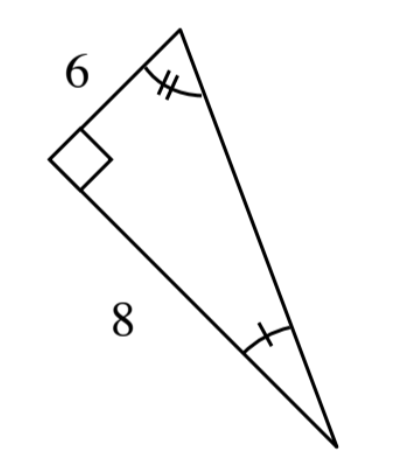### Home > INT2 > Chapter 2 > Lesson 2.2.2 > Problem2-62

2-62.

The ratios Casey wrote from part (a) of problem 2-58 are ratios of the lengths of corresponding sides of the two shapes.

1. Look at the similar triangles below. Which sides correspond? Write ratios for the corresponding side lengths.The sides labeled $15$ and $6$ are corresponding, as are the sides labeled $20$ and $8$.

2. Calculate the length of the hypotenuse of each triangle. Is the ratio of the hypotenuses equal to the ratios you found in part (a)?

Use this method to find the hypotenuse of the second triangle.
Is the ratio of the hypotenuses equal to the ratios you wrote in part (a)?

Use the Pythagorean Theorem to solve for the hypotenuse.

$15^2 + 20^2 = c^2$

$625 = c^2$

$\sqrt{625} = \sqrt{c^2}$

$c = 25$
(Note: the second solution $-25$ is rejected for this problem.)

3. Describe a sequence of transformations that would map one triangle onto the other.